# Cross Multiplication Method

Go back to  'Pair of Linear Equations in Two Variables'

If you learn to use it properly, the cross-multiplication technique will generally prove to be the fastest method to determine the solution to a pair of linear equations. There is no new concept involved in this technique, but it generalizes the solution-finding process in a very convenient way. To understand this technique, consider an arbitrary pair of linear equations (that is, with any coefficients):

\begin{align}& {a_1}x + {b_1}y + {c_1} = 0\\&{a_2}x + {b_2}y + {c_2} = 0\end{align}

Let us eliminate the variable y to find out x:

\begin{align}&\left\{ \begin{array}{l}{b_2} \times \left( {{a_1}x + {b_1}y + {c_1} = 0} \right)\\{b_1} \times \left( {{a_2}x + {b_2}y + {c_2} = 0} \right)\end{array} \right.\\&\Rightarrow \left\{ \begin{array}{l}\,\,\,\,\,{a_1}{b_2}x + {b_1}{b_2}y + {b_2}{c_1} = 0\\\,\,\,\,\,{a_2}{b_1}x + {b_1}{b_2}y + {b_1}{c_2} = 0\\\underline { - \,\,\,\,\,\,\,\,\,\,\,\,\,\,\,\,\,\,\,\,\,\,\,\,\,\,\,\,\,\,\,\,\,\,\,\,\,\,\,\,\,\,} \\\,\,\left( {{a_1}{b_2} - {a_2}{b_1}} \right)x + 0 + {b_2}{c_1} - {b_1}{c_2} = 0\end{array} \right.\\&\Rightarrow \;\;\;x = \frac{{{b_1}{c_2} - {b_2}{c_1}}}{{{a_1}{b_2} - {a_2}{b_1}}}\end{align}

Now, returning to the original pair of equations, let us eliminate x to find out y:

\begin{align}&\left\{ \begin{array}&{a_2} \times \left( {{a_1}x + {b_1}y + {c_1} = 0} \right)\\{a_1} \times \left( {{a_2}x + {b_2}y + {c_2} = 0} \right)\end{array} \right.\\ &\Rightarrow \;\;\;\left\{ \begin{array}{l}{a_1}{a_2}x + {a_2}{b_1}y + {a_2}{c_1} = 0\\{a_1}{a_2}x + {a_1}{b_2}y + {a_1}{c_2} = 0\\\underline { - \,\,\,\,\,\,\,\,\,\,\,\,\,\,\,\,\,\,\,\,\,\,\,\,\,\,\,\,\,\,\,\,\,\,\,\,\,\,\,\,\,\,\,\,\,\,} \\0 + \left( {{a_2}{b_1} - {a_1}{b_2}} \right)y + {a_2}{c_1} - {a_1}{c_2} = 0\end{array} \right.\\ &\Rightarrow \;\;\;\;y = \frac{{{a_1}{c_2} - {a_2}{c_1}}}{{{a_2}{b_1} - {a_1}{b_2}}}\\\;\;\;\;\;\;\;\;\;\; &\qquad\quad= - \left( {\frac{{{a_1}{c_2} - {a_2}{c_2}}}{{{a_1}{b_2} - {a_2}{b_1}}}} \right)\end{align}

Thus, the solution to the pair of linear equations is:

$x = \frac{{{b_1}{c_2} - {b_2}{c_1}}}{{{a_1}{b_2} - {a_2}{b_1}}},\;y = \frac{{ - \left( {{a_1}{c_2} - {a_2}{c_2}} \right)}}{{{a_1}{b_2} - {a_2}{b_1}}}$

Note that we have written the expressions so that the denominators are the same. Now, we do a slight algebraic manipulation, and write this as follows:

\left\{ \begin{align}{l} \frac{x}{{{b_1}{c_2} - {b_2}{c_1}}} = \frac{1}{{{a_1}{b_2} - {a_2}{b_1}}}\\ \frac{{ - y}}{{{a_1}{c_2} - {a_2}{c_1}}} = \frac{1}{{{a_1}{b_2} - {a_2}{b_1}}} \end{align} \right.

$\Rightarrow\;\;\; \fbox{\displaystyle {\frac{x}{{{b_1}{c_2} - {b_2}{c_1}}} = \frac{{ - y}}{{{a_1}{c_2} - {a_2}{c_1}}} = \frac{1}{{{a_1}{b_2} - {a_2}{b_1}}}}}$

This equality then gives us the complete solution – both x and y.

Now comes the cross-multiplication part – it is a way to help us use the above equality easily, without having to remember it. There are three things in the equality – the expression below x, the expression below negative y, and the expression below 1. Given any pair of linear equations, how can we write these expressions even if we do not remember them (and without solving the pair all over again)?

The answer is straightforward. Let us write down the coefficients in the original pair of equations as follows, in a grid fashion:

$\begin{array}{l}{a_1} & {b_1} & {c_1}\\{a_2} & {b_2} & {c_2}\end{array}$

In the first column, we have the coefficients of x. In the second, we have the coefficients of y. And in the third column, we have the constant terms. Now, consider the expression below x in our solution equality: it is $${b_1}{c_2} - {b_2}{c_1}$$. How do we write this? We simply ignore the coefficients of x in our grid, and cross-multiply the coefficients in the remaining two columns, and subtract them: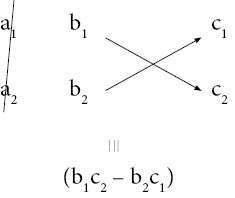Thus, the first part of our solution equality becomes:

$\frac{x}{{{b_1}{c_2} - {b_2}{c_1}}}$

Next, we consider the expression below negative y, which is $${a_1}{c_2} - {a_2}{c_1}$$. To write this, we ignore the column of y coefficients, and cross-multiply the coefficients in the remaining two columns, and subtract them: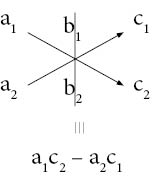Thus, the second part of our solution equality becomes:

$\frac{{ - y}}{{{a_1}{c_2} - {a_2}{c_1}}}$

Finally, we consider the expression below 1, which is $${a_1}{b_2} - {a_2}{b_1}$$. To write this, we ignore the column of constants, and cross-multiply the coefficients in the remaining two columns, and subtract them: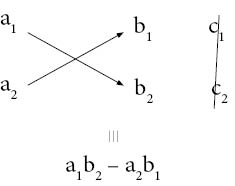Thus, the last part of our solution equality becomes:

$\frac{1}{{{a_1}{b_2} - {a_2}{b_1}}}$

Combining all the three parts, our complete solution to the pair of linear equations becomes:

$\frac{x}{{{b_1}{c_2} - {b_2}{c_1}}} = \frac{{ - y}}{{{a_1}{c_2} - {a_2}{c_1}}} = \frac{1}{{{a_1}{b_2} - {a_2}{b_1}}}$

Easy, isn’t it? Here is an example. Suppose that we have to solve the following pair of equations:

$\begin{array}{l}2x + 3y - 11 = 0\\3x + 2y - 9 = 0\end{array}$

Our solution equality will be of the following form, where we have to figure out the question marks:

$\frac{x}{?} = \frac{{ - y}}{?} = \frac{1}{?}$

To figure out the term below x, we do: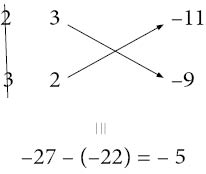Thus, the first part of the solution equality is: $$\frac{x}{{ - 5}}$$. Next, to figure out the term below negative y, we do: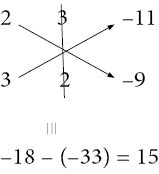Thus, the second part of the solution equality is: $$\frac{{ - y}}{{15}}$$. Finally, to figure out the term below 1, we do: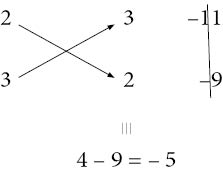Thus, the last part of the solution equality is:$$\frac{1}{{ - 5}}$$. Combining all the three, our complete solution is:

\begin{align}&\frac{x}{{ - 5}} = \frac{{ - y}}{{15}} = \frac{1}{{ - 5}}\\ &\Rightarrow \;\;\;\left\{ \begin{gathered}\!\!\!\!\!\!\!\!\!\!\!x = \frac{{ - 5}}{{ - 5}} = 1\\y = - \left( {\frac{{15}}{{ - 5}}} \right) = 3\end{gathered} \right.\\ &\Rightarrow \;\;\;x = 1,\;y = 3\end{align}

You can verify by substitution or elimination that this is indeed the correct solution.

Example 1: Solve the following pair of linear equations by cross-multiplication:

$\begin{array}{l}2x + 5y - 52 = 0\\3x - 4y + 14 = 0\end{array}$

Solution: The terms below x, negative y and 1 are calculated below: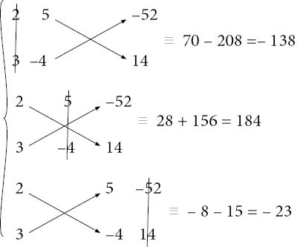Thus, the solution is:

\begin{align}&\frac{x}{{ - 138}} = \frac{{ - y}}{{184}} = \frac{1}{{ - 23}}\\ &\Rightarrow \;\;\;\left\{ \begin{gathered}\!\!\!\!\!\!\!\!\!\!\!\!x = \frac{{ - 138}}{{ - 23}} = 6\\y = - \left( {\frac{{184}}{{ - 23}}} \right) = 8\end{gathered} \right.\\ &\Rightarrow \;\;\;x = 6,\;y = 8\end{align}

Example 2: Solve the following pair of linear equations by cross-multiplication:

$\begin{array}{l}3x + 4y = 32\\6x - 7y = - 11\end{array}$

Solution: First, we rewrite the equations in standard form, that is, in the form $$ax + by + c = 0$$, since it is using this form that we developed our expressions to be used in the cross-multiplication technique:

$\begin{array}{l}3x + 4y - 32 = 0\\6x - 7y + 11 = 0\end{array}$

Now, we calculate the terms below x, negative y and 1 which will come in the solution equality: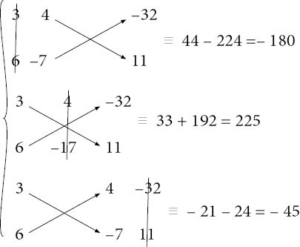Thus, the solution is:

\begin{align}&\frac{x}{{ - 180}} = \frac{{ - y}}{{225}} = \frac{1}{{ - 45}}\\& \Rightarrow \;\;\;\left\{ \begin{gathered}\!\!\!\!\!\!\!\!\!\!x = \frac{{ - 180}}{{ - 45}} = 4\\y = - \left( {\frac{{225}}{{ - 45}}} \right) = 5\end{gathered} \right.\\ &\Rightarrow \;\;\;x = 4,\;y = 5\end{align}

Example 3: Consider the following pair of linear equations:

$\begin{array}{l}{a_1}x + {b_1}y + {c_1} = 0\\{a_2}x + {b_2}y + {c_2} = 0\end{array}$

Suppose that the cross-multiplication technique is applied to solve it. When will the pair turn out to:

1. have a unique solution?

2. be inconsistent?

3. have infinitely many solutions?

Solution: 1. For the pair to have a unique solution, we must have:

$\frac{{{a_1}}}{{{a_2}}} \ne \frac{{{b_1}}}{{{b_2}}}$

Thus,

\begin{align}&{a_1}{b_1} \ne {a_2}{b_1}\\&\Rightarrow \;\;\;{a_1}{b_2} - {a_2}{b_1} \ne 0\end{align}

This means that when we write the solution equality by the cross-multiplication technique, as long as the term below 1 is non-zero, there will be a unique solution:2. To be inconsistent, the slopes of the two lines should be equal, but the y intercepts should be unequal. In terms of coefficients, the required conditions are:

$\frac{{{a_1}}}{{{a_2}}} = \frac{{{b_1}}}{{{b_2}}} \ne \frac{{{c_1}}}{{{c_2}}}$

If you do not remember this, refer again to the discussion where we derived these conditions. Now, these conditions mean that:

$\begin{array}{l}{a_1}{b_2} - {a_2}{b_1} = 0\\{b_1}{c_2} - {b_2}{c_1} \ne 0\\{a_1}{c_2} - {a_2}{c_1} \ne 0\end{array}$

This further means that when we write the solution equality by the cross-multiplication technique, if the term below 1 is zero, and either or both of the terms below x or negative y are non-zero, the pair will be inconsistent:3. To have infinitely many solutions, the condition on the coefficients is:

$\frac{{{a_1}}}{{{a_2}}} = \frac{{{b_1}}}{{{b_2}}} = \frac{{{c_1}}}{{{c_2}}}$

Thus,

$\begin{array}{l}{a_1}{b_2} - {a_2}{b_1} = 0\\{b_1}{c_2} - {b_2}{c_1} = 0\\{a_1}{c_2} - {a_2}{c_1} = 0\end{array}$

This means that when we write the solution equality by the cross-multiplication technique, if all the three terms (below x, below negative y and below 1) are zero, the pair will have infinitely many solutions:Let us apply these facts to some examples.

Example 4: Consider the following pair of linear equations:

$\begin{array}{l}2x + 7y - 1 = 0\\ - 3x + 4y + 3 = 0\end{array}$

Using the cross-multiplication technique, decide whether this pair is consistent or not.

Solution: We have: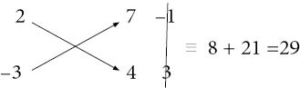Thus, in the solution equality, in the last term, we will have a non-zero denominator, which means that this pair is consistent and has a unique solution.

Example 5: Consider the following pair of linear equations:

$\begin{array}{l}3x - 2y + 1 = 0\\ - 6x + 4y + 7 = 0\end{array}$

Using the cross-multiplication technique, decide whether this pair is consistent or not.

Solution: We have: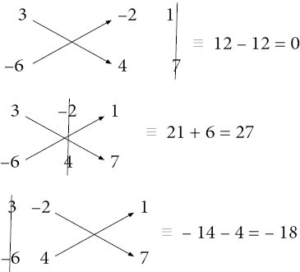Clearly, this pair is inconsistent, because in the solution equality, the denominator in the last term will be zero, but the terms under x and negative y will be non-zero.

Pair of Linear Equations in 2 Variables
Pair of Linear Equations in 2 Variables
grade 10 | Questions Set 2
Pair of Linear Equations in 2 Variables
Pair of Linear Equations in 2 Variables
grade 10 | Questions Set 1